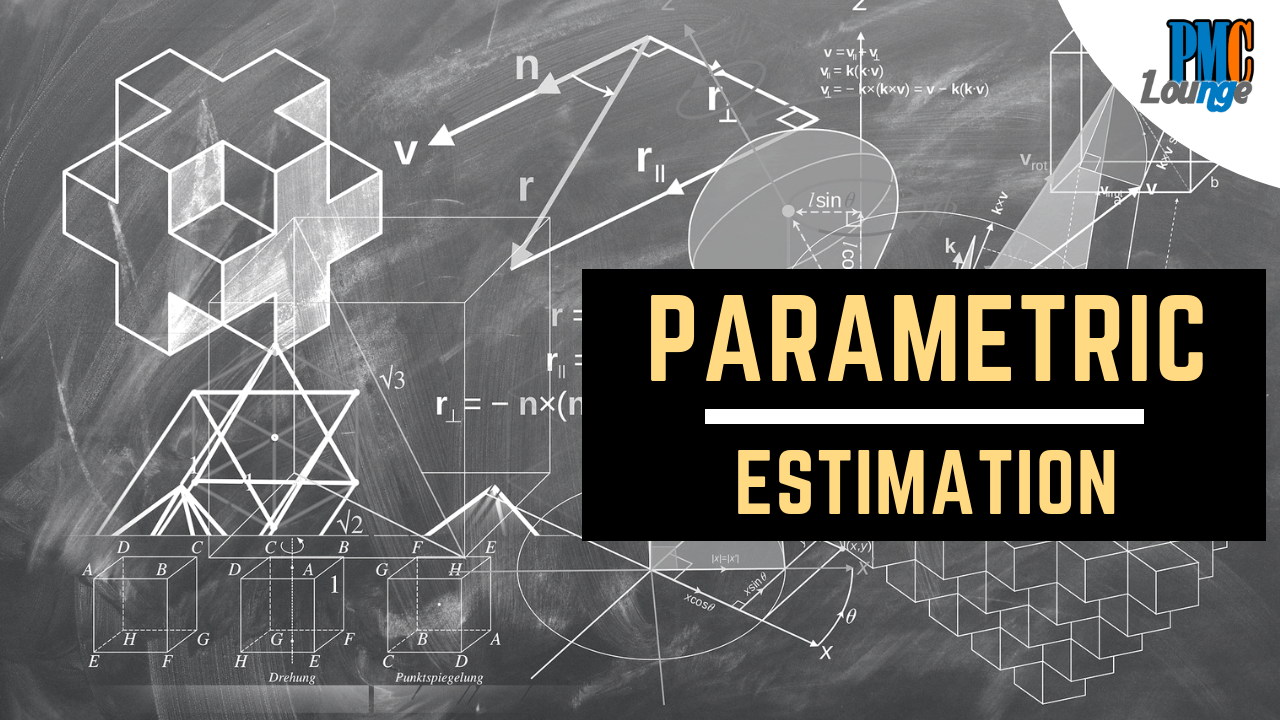Parametric EstimationAs the name suggests, parametric estimates are based on parameters. It is all about using a relationship between these parameters or variables (a unit cost/duration and the number of units) to develop the estimate. Since the focus is on calculations this is a fairly accurate technique. It is used to determine project’s duration and cost.

The variables derived from the project deliverables are plugged into a formula or a software that generates the estimates. This software or formula is based on a database of actual values from past projects or published information. It may sound a bit difficult but is fairly easy to implement.

• First step is to define the specifications of each unit of the deliverable
• Secondly, refer to past projects or research published information (if available) about how many hours of work are required for each unit and how much does it cost

For example, the units could be linear feet of wall surface to paint. This deliverable unit can be further extrapolated for a parametric estimate.

Example of Parametric Estimation using published data

If you have to construct a house, you can consult an estimation publication to find out the cost of construction per square foot. If it is \$200 then you can simply multiply it with the total square foot construction area. Similarly, you can obtain per square foot costs for interiors, painting etc for a final value

Example of Parametric Estimation using historical data

If it historically takes 1 hour to write 50 lines of code and a project module would require about 400 lines of code then it should ideally take 8 hours to finish the job

• Parametric estimates are more credible as these come from sources published by large reputable organizations
• You can also recalculate the planned units of deliverable based on the actual units delivered

• Published data is although readily available but it may not be true for every project
• Parametric estimation is actually less successful with tasks that produce intangible outputs. For instance, using parametric estimation for coding maybe inaccurate as each line of code may require vastly different amounts of thought and creativity

Two Important Concepts with respect to Parametric Estimation

There are two concepts that must be considered about Parametric Estimates,

1. Regression Analysis

Can you consider 2 variables instead of one? In the example above instead of taking 1 hour = 50 lines of code, can you also consider the number of defects and then the time it takes to troubleshoot. Wouldn’t you obtain a more accurate estimate that way?

2. Learning Curve

A human learning curve cannot be ignored. It may take 8 hours to finish 400 lines of code but if the programmer has written similar codes 25 times already, don’t you think it should take less than 8 hours to write the 400 lines of code?

Check more articles on Schedule Management